top of page
Search
• otw

It has become clear from some of your questions that quite a few of you are unfamiliar with the hexadecimal system, or simply, hex. The hexadecimal system is used throughout computing and if you have never studied this Base16 numering system, it may appear relatively opaque.

For those of you who may have been overwhelmed by this seemingly mysterious numbering system, I dedicate this brief introduction and overview of the hexadecimal numbering system.

As you already know, you encounter the hexadecimal system throughout your computer and software. It usually looks some thing like this 0x4D or simply 4D. Immediately, you recognize something is different here because first, it often starts with that strange 0x, and second, there are letters in there and not just the digits (0-9).

We will often encounter hex in hard drive images such as in digital forensics such as below.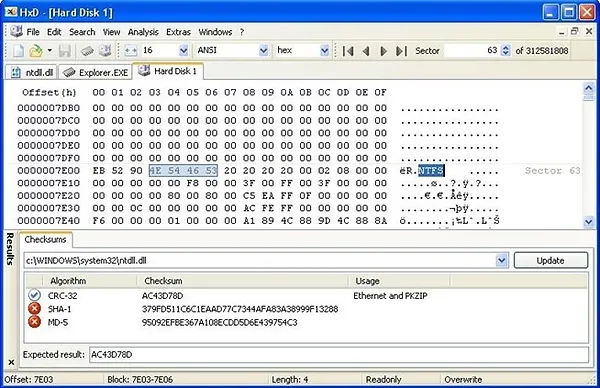Or we might also encounter hex while using Wireshark, like below.And we are very likely to encounter hex in reading our IDS alerts such as in Snort, like that below.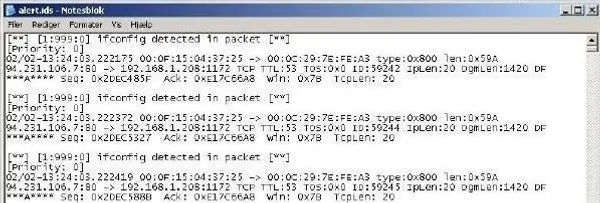Among the most important reasons to understand and study hex is that our MAC addresses on all NICs and network devices are all in hex, and IPv6 will ALL be in hex, once it is widely adopted.If you are not familiar with hex, this is the time to take a few minutes to try to understand it. A few minutes now will likely save you hours of headaches later.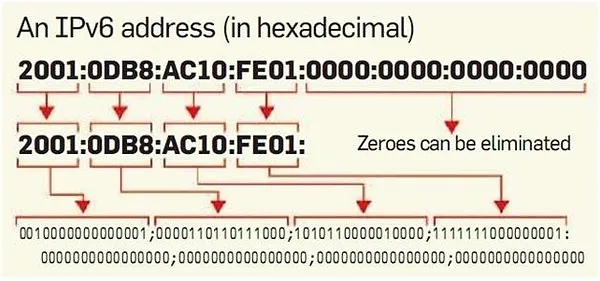## ​

One of the best ways to come to understand the Base16 or hexadecimal system is to go back to the basics of a numbering system you are very familiar with, the Base10, or decimal system. In that system, we have 10 digits (0-9) and each column in that system represents a value 10 times greater than the one to the right of it. So, for instance:

31337

Represents (starting on the right and moving left):

7 ones (1) 3 tens (10) 3 hundreds (100) 1 thousand (1000) 3 ten thousands (10,000)

Each place holder is 10 times larger than the previous one. The hex system works similarly, but each column is 16 times larger than the previous column. So, the first four hex columns have values like this:

4096 256 16 1

Each is 16 times greater than the column to its right.

## ​

The hex system is a base16 numbering system. Like the decimal or Base10 system, the hex system has to have 16 digits to cover all the possibilities. Unfortunately, we only have 10 to work with (0-9), so we need to add six more. The computer industry has decided to use the letters A-F to represent these additional values. This means that the hex system has the following values.

0 = 0 1= 1 2 = 2 3 = 3 4 = 4 5 = 5 6 = 6 7 = 7 8 = 8 9 = 9 A = 10 B = 11 C = 12 D = 13 E = 14 F = 15

This means that when we see a hex representation of a number such as:

0x45

We can evaluate it similarly to a decimal number except the second column represents 16s and not 10s. This means that 0x45 in hex can be evaluated such as:

5 x 1 = 5 4 x 16 = 64 --------------- = 69

If we have a hex number such as DE, we can calculate its value this way:

D represents 13 x 16 = 208 E represents 14 x 1 = 14 -------------------------------------- = 222

## ​

In computing, a bit is a single on/off switch often represented by a 0 or a 1. A byte is eight bits often represented by 0000 0000. A byte is an important unit in computing as it often used to represent an ASCII character or a single octet in an IP address, among many other things. The range of numbers that one byte can represent is 0-255 or 256 values. That is why in our IPv4 addresses, each octet (byte) has a value from 0-255, such 192.168.0.254 with a netmask of 255.255.255.0. Follow me?

Most humans have a hard time working in the Base2 system that underlies our computer systems. Base2 numbers might be represented like this:

1101 1100

Athough this number represents 2220 in decimal, the conversion to decimal is not simple or intutive.

The hex system allows us to represent the values of a single byte with just two digits as the two digit hex values also range from 0 - 255. Hex is more compact than the Base2 system and it looks and acts a lot more like the decimal system than Base2. In addition, every value that can be represented by a byte can be represented in hex by the values between 0x00 - 0xFF (255).

## ​

Probably the easiest way to do hex math is to use the calculator built into every Windows system. Open the Windows calculator like below:​Now, go to the View menu at the top and choose "Programmer".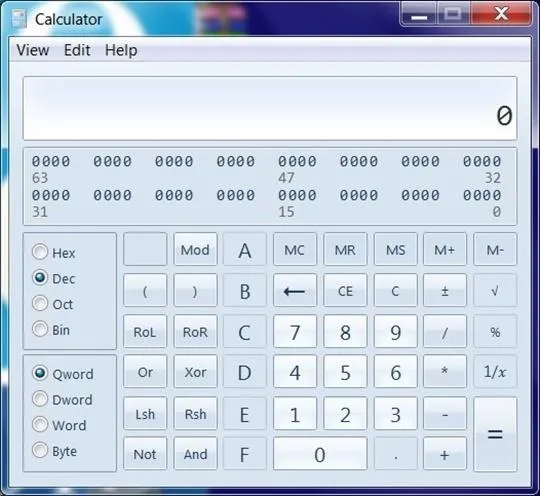As you can see on the left side of the calculator about midway down, there are four buttons, Hex, Dec, Oct, Bin. By default, it should be set to Dec or Decimal, our familiar Base10 system. If I want to enter a hexadecimal number, simply click on Hex and then enter the number.After I do so, I can then toggle to the Dec button and it will convert my hexadecimal number to a decimal number.Finally, if we want to add, subtract, multiply or divide in hex, we can use the Programmer view in the Calculator as well. That is, is we wanted to add the hex value DE to the hex value A5, we simply enter DE in the calculator press the addition (+) and then enter the A5 and then press equal. The result will be 183 in hex.​And then we can toggle to the Dec button and get the decimal equivalent or 387.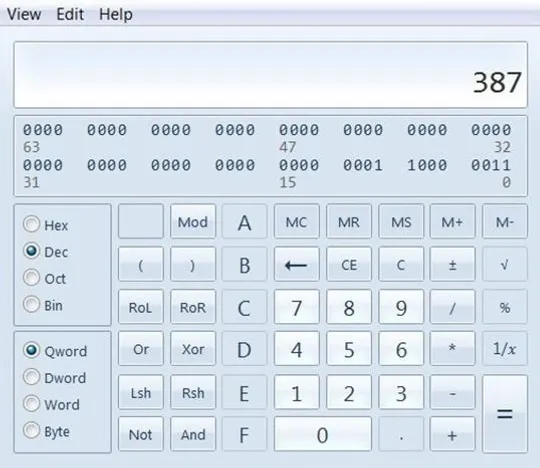​Hope this brief tutorial on hex is helpful!

2,176 views
bottom of page